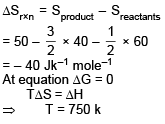Courses

# MCQ (Practice) - Thermodynamics (Level 1) Part -1

## 30 Questions MCQ Test | MCQ (Practice) - Thermodynamics (Level 1) Part -1

Description
This mock test of MCQ (Practice) - Thermodynamics (Level 1) Part -1 for Class 11 helps you for every Class 11 entrance exam. This contains 30 Multiple Choice Questions for Class 11 MCQ (Practice) - Thermodynamics (Level 1) Part -1 (mcq) to study with solutions a complete question bank. The solved questions answers in this MCQ (Practice) - Thermodynamics (Level 1) Part -1 quiz give you a good mix of easy questions and tough questions. Class 11 students definitely take this MCQ (Practice) - Thermodynamics (Level 1) Part -1 exercise for a better result in the exam. You can find other MCQ (Practice) - Thermodynamics (Level 1) Part -1 extra questions, long questions & short questions for Class 11 on EduRev as well by searching above.
QUESTION: 1

Solution:
QUESTION: 2

### Consider the reaction at 300 K H2(g) + Cl2(g)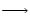2HCl(g) ΔH° = -185 kJ If 3 mole of H2 completely react with 3 mole of Cl2 to form HCl. What is ΔU° for this reaction ?

Solution:

For 3 moles of Cl2 & HCl
ΔU = – 3 × 185 = – 555 kJ

QUESTION: 3

### Ethyl chloride (C2H5Cl), is prepared by reaction of ethylene with hydrogen chloride : C2H4(g) + HCl (g) → C2H5cl(g) ΔH = -72.3 kJ What is the value of ΔE (in kJ), if 70 g of ethylene and 73 g of HCl are allowed to react at 300 K.

Solution:

2 mole HCl is consumed completely for 1 mole consumption of HCl
ΔU = ΔH – Δng RT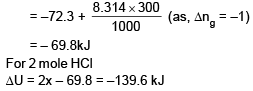QUESTION: 4

Based on the first law of thermodynamics, which one of the following is correct?

Solution:
QUESTION: 5

One mole of ideal gas is allowed to expand reversibly and adiabatically from a temperature of 27°C. If the work done by the gas in the process is 3 kJ, the final temperature will be equal to (Cv = 20 J/K mol)

Solution:

w = nCv ΔT
⇒ –3 × 1000 = 1 × 20 × (T – 300)
T = 150 K

QUESTION: 6

What is the change in internal energy when a gas contracts from 377 ml to 177 ml under a constant pressure of 1520 torr, while at the same time being cooled by removing 124 J heat ? [Take : (1 L atm) = 100 J]

Solution:

ΔU = Q + w
Q = –124 J
W = –Pext(v2 – v1)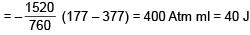ΔU = –124 + 40 = – 84 J

QUESTION: 7

The heat capacity of liquid water is 75.6 J/mol K, while the enthalpy of fusion of ice is 6.0 kJ/mol. What is the smallest number of ice cubes at 0°C, each containing 9.0 g of water, needed to cool 500 g of liquid water from 20°C to 0°C?

Solution:

Heat released  by 500 gm of liquid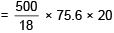Heat absorbed by 'n' no of ice each 9 gm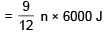Heat absorbed = Heat released
Which gives n = 14

QUESTION: 8

An ideal gas is taken around the cycle ABCDA as shown in figure. The net work done during the cycle is equal to :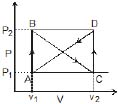Solution:

Area of ΔABC = – Area of ΔACD

QUESTION: 9

Molar heat capacity of water in equilibrium with ice at costant pressure is

Solution: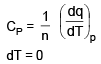QUESTION: 10

A cyclic process ABCD is shown in PV diagram for an ideal gas. Which of the following diagram represents the same process ?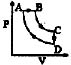Solution:
QUESTION: 11

A diatomic ideal gas initially at 273 K is given 100 cal heat due to which system did 209 J work. Molar heat capcity (Cm) of gas for the process is :

Solution: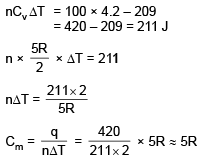QUESTION: 12

One mole of an ideal monoatomic gas expanded irreversibly in two stage expansion.

State-1 (8.0 bar, 4.0 litre, 300 K)

State-2 (2.0 bar, 16 litre, 300 K)

State-3 (1.0 bar, 32 litre, 300 K)

Total heat absorbed by the gas in the process is :

Solution: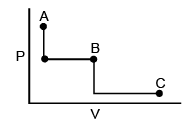Total work done = – 2(16 – 4) – 1 (32 – 16)
= –24 – 16 = – 40 bar litre
= – 4000 J
q = – w = 4000 J

QUESTION: 13

For an ideal monoatomic gas during any process T = kV, find out the molar heat capacity of the gas during the process. (Assume vibrational degree of freedom to be active)

Solution:
QUESTION: 14

A gas (Cv,m =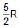) behaving ideally was allowed to expand reversibly and adiabaticaly from 1 litre to 32 litre. It's initial temperature was 327°C. The molar enthalpy change (in J/mole) for the process is

Solution: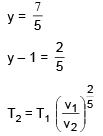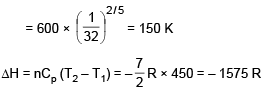QUESTION: 15

The bond energy (in kcal mol–1) of a C—C single bond is approximately

Solution:
QUESTION: 16

Two moles of an ideal gas (Cv =) was compressed adiabatically against constant pressure of 2 atm. Which was initially at 350 K and 1 atm pressure. The work involve in the process is equal to

Solution: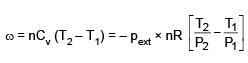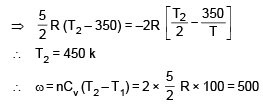QUESTION: 17

An insulated container of gas has two chambers separated by an insulating partition. One of the chambers has volume V1 and contains ideal gas at pressure P1 and temperature T1. The other chambers has volume v2 and contains ideal gas at pressure Pand temperature T2. If the partion is removed without doing any work on the gas, the final equilibrium temperature of the gas in the container will be

Solution: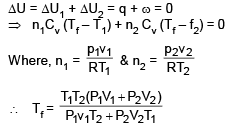QUESTION: 18

The maximum efficiency of a heat engine operating between 100°C and 25°C is

Solution: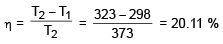QUESTION: 19

A heat engine operating between 227°C and 27°C absorbs 2 Kcal of heat from the 227°C reservoir reversibly per cycle. The amount of work done in one cycle is :

Solution: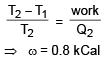QUESTION: 20

A reversible heat engine A (based on carnot cycle) absorbs heat from a reservoir at 1000 K and rejects heat to a reservoir at T2. A second reversible engine B absorbs, the same amount of heat as rejected by the engine A, from the reservoir at T2 and rejects energy to a reservoir at 360 K. If the efficiencies of engines A and B are the same then the temperautre T2 is

Solution: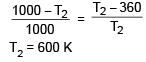QUESTION: 21

For the reaction at 300 K

A(g) + B(g) → C(g) DE = -3.0 kcal ; ΔS = -10.0 cal/K

value of ΔG is

Solution:

ΔG = ΔH – TΔS
Also, ΔH = ΔU + Δng RT
= – 3000 – 1 × 2 × 300
= – 3600 Cal
∴ ΔG = – 3600 + 10 × 300 = – 600 Cal

QUESTION: 22

The entropy change when two moles of ideal monoatomic gas is heated from 200 to 300°C reversibly and isochorically

Solution: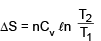QUESTION: 23

What is the free energy change (ΔG) when 1.0 mole of water at 100° C and 1 pressure is converted into steam at 100°C and 1 atm pressure ?

Solution:

The process is at equilibrium

QUESTION: 24

What if the free energy change (ΔG) when 1.0 mole of water at 100°C and 1 atm pressure is converted into steam at 100°C and 2 atm pressure ?

Solution:
QUESTION: 25

What can be concluded about the values of ΔH and ΔS from this graph ?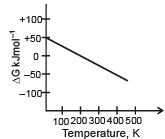Solution:

ΔG = ΔH – TΔS
–ΔS = negative (slope)
∴ ΔS = positive
From graph ΔH > 0

QUESTION: 26

If DHvaporisation of substance X (l) (molar mass : 30 g/mol) is 300 J/g at it's boiling point 300 K, then molar entropy change for revesible condensation process is

Solution: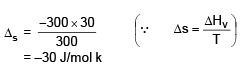QUESTION: 27

Pressure of 10 moles of an ideal gas is changed from 2 atm to 1 at against constant external pressure without change in temperature. If surrounding temperature (300K) and pressure (1 atm) always remains constant then calculate total entropy change (ΔSsystem + ΔSsurrounding) for given process.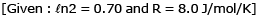Solution: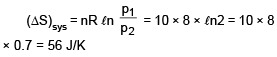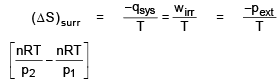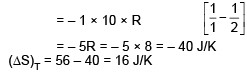QUESTION: 28

The enthalpy of tetramerization of X in gas phase (4X(g) → X4(g)) is _100 kJ/mol at 300 K. The enthalpy of vaporisation for liquid X and X4 are respectively 30 kJ/mol and 72 kJ/mol respectively. ΔS for tetramerization of X in liquid phase is –125 J/K mole at 300 K. What is the ΔG at 300 K for tetramerization of x in liquid phase.

Solution: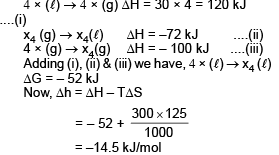QUESTION: 29

The change in entropy of 2 moles of an ideal gas upon iosthermal expansion at 243.6 K from 20 litre until the pressure becomes 1 atm, is :

Solution: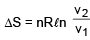QUESTION: 30

Standard entropy of X2, Y2 and XY3 are 60, 40 and 50 JK-1 mol-1, respectively. For the reaction,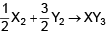, ΔH = –30 kJ to be at equilibrium, the temperature will be

Solution: